# Scattering Parameters Tutorial: What Are They & What Do They Do?

Contents

## What are S Parameters?

The S-parameters, also known as scattering or S-matrix parameters, represent how RF energy moves through a multi-port network. It represents the linear properties of RF electronic components and describes how energy travels through an electric network.

The S-parameter matrix calculates characteristics like gain, loss, impedance, phase group delay, and VSWR. S-parameters are used to represent a complex network as a simple “black box” and clearly indicate what happens to the signal within that network. The black box could contain anything, ranging from a resistor, a transmission line, or an integrated circuit.

When discussing S-parameters, the term “scattering” describes how the travelling currents and voltages in a transmission line are affected when they come into contact with a discontinuity brought on by the introduction of a network into the transmission line.

S parameters are widely employed in electrical, electronic, and communication systems engineering to effectively describe the electrical characteristics of linear electrical networks, particularly those operating at high frequencies and subject to various steady-state input signals with small amplitudes.

S-parameters can be used at any frequency, but they are primarily used in radio frequency (RF) and microwave networks because signal power and energy considerations are simpler to measure than currents and voltages. S-parameter measurements must include frequency information in addition to characteristic impedance or system impedance because S-parameters are frequency-dependent.

A network or circuit interconnects various electrical components such as resistors, inductors, and capacitors. A pair of terminals at which a signal may enter or leave a network is called a Port or any pair of terminals into which energy is supplied or from which energy is withdrawn.

An electrical network, or “black box,” to be characterised using S parameters may have any number, N, of ports, as shown in Figure 1. Ports are the points at which electrical currents either enter or exit the network. Sometimes these are referred to as pairs of “terminals”.

## What Do S-Parameters Indicate?

S-parameters are complex numbers (numbers with real and imaginary parts) that can be used either directly or in a matrix to display the amplitude and/or phase of reflection or transmission characteristics in the frequency domain.

When a complex time-varying signal is passed through a linear network, the amplitude and phase shifts can distort the time-domain waveform dramatically. As a result, amplitude and phase information in the frequency domain are critical. S-parameters are a parameter that supports both information and has many advantages for the characterization of high-frequency devices.

When specifying a set of S-parameters, the following information must be defined:

• The frequency
• The nominal characteristic impedance (often 50 Ω)
• The allocation of port numbers
• Conditions which may affect the network, such as temperature, control voltage, and bias current, where applicable

## The Specifics of S-Parameters

According to the S-parameter approach, an electrical network is a “black box” that contains a variety of interconnected basic electrical circuit components, including resistors, capacitors, inductors, and transistors, and that communicates with other circuits via ports.

The S-parameter matrix, which can be used to determine the network’s response to signals applied to the ports, is a square matrix of complex numbers that serves as a characteristic of the network. An electrical network described by S-parameters may have a number of ports.

S parameters offer a versatile means of expressing various electrical attributes of networks or components, including parameters like gain, return loss, voltage standing wave ratio (VSWR), network stability, and reflection coefficient.

Since S parameters exhibit variations with frequency, it is imperative to incorporate frequency dependence when characterising a network using S-parameters, along with considering its characteristic impedance or system impedance.

## The Scattering (S) Matrix

The scattering (S) matrix describing an N-port network contains N2 elements or S parameter coefficients, each representing a possible input-output interaction. S-parameters are readily represented in matrix form and obey the rules of matrix algebra. The number of rows and columns is equal to the number of ports.

For the S-parameter Sij, the j subscript represents the excited port (the input port), and the i subscript represents the output port. The first number following the “S” in S-parameters is the port where the signal emerges, and the second number is the port where the signal is applied. The scattering (S) matrix relates the scattered waves b1 and b2 to the incident waves a1 and a2 as shown in Figure 2.

Scattering Matrix S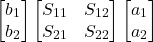Thus, the scattering (S) matrix for a two-port network is the 2 × 2 matrix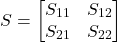The S-parameter matrix is fundamental for two-port networks and forms the foundation for creating higher-order matrices in larger networks.

Parameters along the leading diagonal of the S-matrix, S22 and S11, are referred to as
reflection coefficients because they refer to the reflection occurring at one port only.
Off-diagonal S parameters, S12 and S21, are referred to as transmission coefficients because they refer to what happens from one port to another.

It should be noted that each S-parameter is a complex number. So, if actual data were presented in matrix format, each Sij would have a magnitude and phase angle. The most common “N-port” networks in microwaves are one-port and two-port networks.

A one-port network is an electrical system with two terminals: current enters through one and exits through the other. Resistors, inductors, and capacitors are examples of one-port networks because each one has two terminals.

The S-parameter matrix for a network with just one port will have just one element represented in the form Snn, where n is the number allocated to the port. A one-port network representation is shown in the following Figure 3.

A port is represented by the pair of terminals 1 and 1′. In this case, we have only one port since it is a one-port network.

Similarly, a two-port network is a pair of two-terminal electrical networks in which current enters through one terminal of each port and exits through another terminal of the same port.

The S-parameter matrix shown in figure 4, is foundational for two-port networks, paving the way for matrices in larger networks.

In this case, one pair of terminals, 1 & 1′, represents port 1, and the other pair of terminals, 2 & 2′, represents another port 2. As shown in the figure, a two-port network has four variables: V1, V2, I1, and I2. We can choose two variables to be independent and two variables to be dependent. As a result, we will have six possible pairs of equations.

These equations represent the dependent variables in terms of independent variables. The coefficients of independent variables are called parameters. So, each pair of equations will give a set of four parameters.

## S-Parameter For Two-Port Network

Consider a two-port network. The signal at a port, say port 1, can be thought of as the superposition of two waves travelling in opposite directions. Each port is depicted as two nodes by convention in order to assign a name and value to these opposing direction waves as shown in Figure 5.

The variable ai represents the incident wave to port i and the variable bj represents the reflected wave from port j. The magnitudes of the ai and bj variables are voltage-like variables. For a two-port device, a four-element matrix of S-parameter data represents the network’s bidirectional behaviour as a function of frequency as shown in the figure.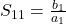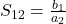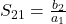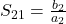The above equations for S11 and S21 are derived from network analysis or measurements by setting the value of the incident signal a2=0 and solving for the above S-parameter ratios as a function of a1. Similarly, S12 and S22 are derived by setting the value of a1=0 and solving for the other ratios.

In this example, S11 is defined as the ratio of the reflected wave to the incident wave at port 1, while port 2 is terminated on the characteristic impedance. The value of S11 provides a direct measure of the port matching condition; that is, S11 = 1 represents an open circuit; S11 = -1 represents a short circuit; and S11 = 0 represents a perfectly matched circuit.

Thus, for a two-port network, the S-parameters are given by,

(1)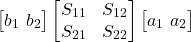(2)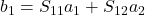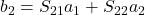To measure S11, we send a signal to port one and measure the reflected signal. In this case, no signal is injected into port 2, so a2=0; we only inject one signal at a time for almost all laboratory S-parameter measurements. To measure S21, we inject a signal through port 1 and measure the resulting signal power through port 2. S12 involves injecting a signal into port 2 and measuring the signal power leaving port 1, whereas S22 involves injecting a signal into port 2 and measuring the reflected signal.

## What is an RF Transmission Line?

In general, a transmission line is a medium or structure that creates a path for energy to be transferred from one location to another. In RF engineering, transmission lines are specialised cables and waveguides designed to carry alternating current (AC) and radio-frequency electromagnetic waves.

RF transmission lines “enclose” electromagnetic waves, preventing them from escaping and causing power loss. Transmission lines use specialised construction techniques such as precise conductor dimensions and spacing, as well as impedance matching, to carry electromagnetic signals with the least amount of reflection and power losses.

## Properties of Transmission Lines

A transmission line can be represented mathematically as a two-port network. For simple lines, the network is assumed to be linear, and the two ports are interchangeable. If the line’s behaviour is uniform along its length, it can be described largely by a single parameter: the characteristic impedance Zc, which is the ratio of the complex voltage of a given wave to the complex current of the same wave at any point along the line.

## S- Parameters of a Transmission Lines

A transmission line is an example of a two-port symmetrical network. The transmission properties will not change if port one and port two are switched. Transmission lines in RF circuits are laid with exact dimensions, thickness, spacing, and impedance matching to transmit electromagnetic signals with the least amount of reflection and power loss.

Two-port network modelling is used in transmission line analysis. Transmission line scattering parameters (S-parameters) are similar to the family of parameters. S-parameter measurements are frequent in RF and microwave systems.

Network analyzers can be used to directly measure S-parameters. In an S-parameter synthesis, incident and reflected waves replace system inputs and outputs. This method is ideal for high-frequency RF and microwave applications.

Transmission lines in RF circuits that connect various components can be represented as two-port networks with two incident waves, a1 and a2 in ports one and two. The variables b1 and b2 represent the reflected waves in ports one and two, respectively.

The incident and reflected waves are typically normalized with the characteristic impedance value. The general S-parameters of a two-port transmission line model are as follows:

(3)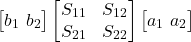There are no reflections in the transmission line when the incident wave at port one and the output are matched, so a2 becomes zero. Additionally:

• S11 = input port reflection
• S12 = reverse gain
• S21 = forward gain (linear gain/insertion loss)
• S22 = output port reflection

## Lossy and Lossless Transmission Line S-Parameters

A transmission line is an example of a two-port symmetrical network. Asymmetrical networks’ S-parameters have a unique relationship: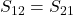Changing ports one and two has no effect on the transmission line’s S-parameter properties. The following factors can affect transmission line S-parameters:

• Line Geometry
• Characteristic impedance
• Frequency

The S-parameters and transmission line properties change when any of these factors change. The characteristic impedance Zc and propagation constant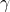influence transmission line S-parameters. As the transmission line is symmetrical and reciprocal, S11=S22 and S12=S21.

The following equation gives the propagation constantand characteristic impedance Zc,

A small section of a transmission line can be analyzed using a lumped-circuit analogy. A unit-length-long piece of the line is represented by a series inductor L of resistance R, and a parallel capacitor C with inductance G are the distributed elements of the transmission line of length L.

The inductor L is a current-carrying conductor that creates a magnetic field around itself to delay the voltage. The resistance R represents the conductor’s ohmic or resistive losses. The impedance is formed by these two parameters: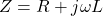Because of the finite distance between two conductors, the capacitor C exists. Finally, the dielectric losses in a dielectric medium that fills the space between two conductors are represented by the conductance G. The admittance is determined by these two parameters: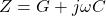Two parameters can be derived using the impedance and admittance:

• The propagation constant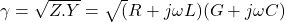• The characteristic impedance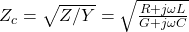## Which S-Parameter is Equivalent to Forward Gain?

S21 refers to the signal exiting at Port 2 for the signal incident at Port 1 expressed as the
ratio of the two waves, b2 and a2.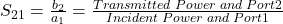For a circuit or system designer, the utmost significance of S21 lies in its ability to provide insights into the anticipated frequency-dependent transmission gain or loss at a specific frequency.

## Which S-Parameter is Equivalent to Input Return Loss?

S11 refers to the signal reflected at Port 1 for the signal incident at Port 1 expressed as the
ratio of the two waves, b1 and a1.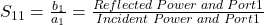Engineers commonly rely on S11 when assessing the quality of electrically compact structures like connectors and vias, which serve as impedance discontinuities. When crafting a transmission path, a lower return loss is preferred because it signifies that fewer energy reflections occur as a result of impedance mismatches and associated disruptions to the energy flow.

## Which S-Parameter is Equivalent to Output Return Loss?

Output return loss S22 shares a parallel definition with input return loss, but it pertains to the output port (Port 2) rather than the input port. It is quantified as the ratio between the two waves, b2 and a2.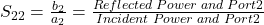## Which S-Parameter is Equivalent to Reverse Gain and Reverse Isolation?

S12 refers to the signal exiting at Port 1 for the signal incident at Port 2 expressed as the
ratio of the two waves, b1 and a2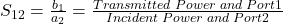For circuit and system designers, the primary significance of S21 lies in its ability to convey the expected degree of frequency-dependent forward transmission gain or loss at a specific frequency.